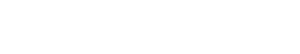# Percentile

Percentile is a metric of centrality. It’s used to determine the value below which a specified proportion of observations in a dataset fall. The weighted sum of the ranks of all values in the dataset is used to compute percentile.

Percentile is closely related to the term “percentile rank,” which is used to describe the percentage of values in a dataset that is equal to or less than a given value. Percentile rank is computed using the same formula as percentile, but instead of summing the ranks of all values in the dataset, only the rank of the given value is considered.

Outside of text analytics, percentile may be used to describe the value below which a specified proportion of a population falls. In this context, the term “percentile rank” is used to describe the percentage of the population that is equal to or less than a given value.

While both percentile and percentile rank are measures of centrality, they are not interchangeable. It’s important to be clear about which metric you’re using when communicating your results.

In the context of text analytics, percentile is a more accurate measure of centrality than percentile rank. This is because the weighted sum of the ranks of all values in the dataset provides a more granular view of where a given value falls in relation to all other values in the dataset.

When comparing percentile to other terms, it is important to note that percentile is a metric of centrality, while terms like median and mode are measures of dispersion. This means that percentile can be used to compare values within a dataset, while median and mode cannot.

## When to use percentile?

There are a few different situations where you might want to use percentile:

• To compare values within a dataset: If you want to know how a given value compares to all other values in the dataset, percentile is the metric for you.
• To understand the distribution of values in a dataset: Percentile can be used to get a quick understanding of the distribution of values in a dataset.
• To find outliers in a dataset: Percentile can be used to identify outliers in a dataset. Values that fall far below or above the rest of the data are likely to be outliers.

## When not to use percentile?

There are also a few situations where you might not want to use percentile:

• To compare values across different datasets: Because percentile is a metric of centrality, it can only be used to compare values within a single dataset. If you want to compare values across different datasets, you’ll need to use a different metric.
• To understand the dispersion of values in a dataset: Percentile is a metric of centrality, not dispersion. If you want to understand the dispersion of values in a dataset, you’ll need to use a different metric like median or mode.
• To find the most common value in a dataset: Again, because percentile is a metric of centrality, it can’t be used to find the most common value in a dataset. If you want to find the most common value, you’ll need to use a different metric like mode.Unlock the power of actionable insights with AI-based natural language processing.